# Electronics and Communication Engineering - Electromagnetic Field Theory

11.

A line of characteristic impedance z0 ohms, phase velocity VP = 2 x 108 m/sec and length l = 2 m is terminated by a load impedance zL Ohms. The reflection coefficients at the input end and load end are respectively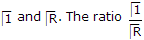for a frequency of 50 MHz will be

 A. 1 B. - 1 C. -1 D. 2

Explanation:

ri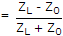.

12.

When a particular mode is excited in a waveguide, these appears an extra electric components in the direction of propagation. The resulting mode is

 A. Transverse electric B. Transverse magnetic C. Longitudinal D. Transverse Electromagnetic

Explanation:

No answer description available for this question. Let us discuss.

13.

An antenna has a gain of 44 dB. Assuming that the main beam of the antenna is circular in cross-section the beamwidth will be

 A. 0.4456° B. 1.4456° C. 2.4456° D. 3.4456°

Explanation: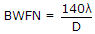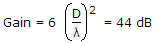.

14.

The directive gain of an antenna is given by

 A.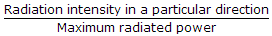B.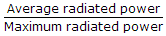C.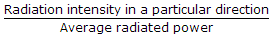D.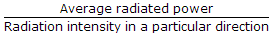Explanation:

No answer description available for this question. Let us discuss.

15.

In cellphone communication base antennas are connected to other base antennas in

 A. line of sight communication B. ionospheric propagation C. ground wave propagation D. medium wave propagation